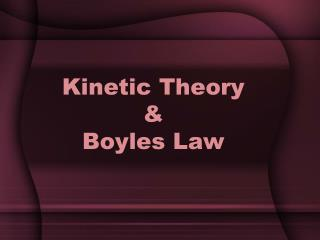DownloadDownload PresentationKinetic Theory & Boyles Law

# Kinetic Theory & Boyles Law

Télécharger la présentation## Kinetic Theory & Boyles Law

- - - - - - - - - - - - - - - - - - - - - - - - - - - E N D - - - - - - - - - - - - - - - - - - - - - - - - - - -

1. ### Kinetic Theory & Boyles Law

2. Kinetic Theory of Gases All matter consists of tiny particles in constant motion Kinetic Energy – energy an object has due to it’s motion.
3. Assumptions Particles are small, hard spheres with insignificant volume Particle motion is rapid, constant & random Move in straight path until collision occurs All collisions btwn particles are ELASTIC Kinetic energy is transferred with no loss (so total kinetic energy stays constant)
4. Gas Pressure Due to simultaneous collisions of billions of particles of gas on a object UNITS: Pascals (Pa) (SI) Standard atmosphere (atm) Millimeters of mercury (mmHg) 1atm = 760mm Hg = 101.3 kPa
5. Atmospheric Pressure Results from collisions of atoms & molecules in the air with objects As you increase altitude the atm pressure decreases b/c density decreases
6. Gases & Temperature Heated particles store energy Causes particles to move faster! Causes kinetic energy to change, so we use the avg. kinetic energy UNITS: Kelvin Directly proportional to avg KE of particles
7. Gas Property Compressibility Measure of how much the volume of matter decreases under pressure Think of squeezing gases into a smaller container! Easier with gases b/c of the space btwn particles
8. Factors Affecting Gas Pressure Amount of Gas (mol) - More particles, more collisions, more pressure Volume (L) - More volume, less pressure - What happens if you compress a gas? Temperature (K) - increase temp, increase collisions, increase pressure
9. ### Boyle’s Law

Pressure & Volume
10. Boyle’s Law If temperature & mass is constant, as pressure increases the volume decreases. P1V1 = P2V2
11. Example Problem Nitrous oxide (N2O) is used as an anesthetic. The pressure on 2.5L of N2O changes from 105 kPa to 40.5 kPa. If the temperature does not change, what will the new volume be? V1= P1= V2 = P2 =